VKRC Syntax warnings & VASS Standard
What makes our programme really user friendly is the new functionality that shows syntax warnings during program editing.

The user can fix quickly and automatically some common syntax warnings for the whole program. With one double click on the left margin icon idicating the particular syntax warning, the user will be asked if the syntax fixing should be processed automatically for one or all marked commands.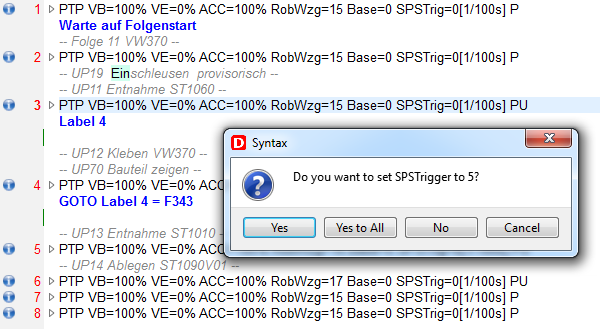All syntax warnings are groupped into the following categories:
•syntax info - it is only a simple message for VKRC command that can be edited and saved correctly but it is not fully compatible with the built-in VKRC standard checker

•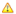syntax warning - this message indicates some unusual syntax - it can be edited and saved as well

•syntax error - command with this message can not be saved correctly, it will be ommited during saving the programAll messages for the first syntax warnings' category are listed below
• The first and the last point should have equal positions
• The first and the second point should have equal positions
• Only A15 = EIN and SPSMAKRO0 = EIN required for this point
• A15 = EIN in the first subfold required for this point
• SPSMAKRO0 = EIN in the second subfold required for this point

Following commands can the user fix automatically.

• For the first point in Folge max speed is 10%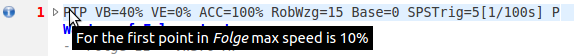• For the first point VE should be 0• For the last point VE should be 0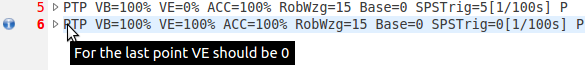• For point with VE=100% SPSTrig should be 0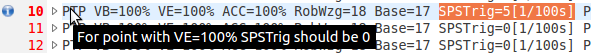• For point with VE=0% SPSTrig should be 5• For point with WARTE BIS subfold Genau should be 0 [mm/s]
• For point with WARTE BIS subfold VE should be 0 %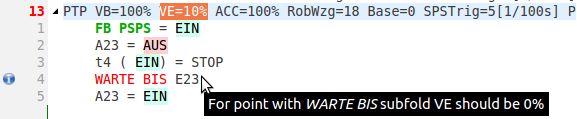• The first command in point should be FB PSPS or FB ONL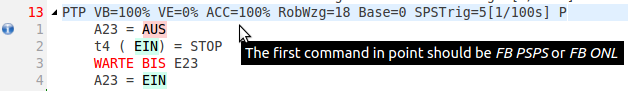The second syntax warnings' type
• The first point in program should be PTP
• The last point in program should be PTP
• This program is NOT registered Tech command will not be saved
• Could not read all parameters for tech command
• + operand in FB PSPS command• Gun 1 balans missing bin2 = ?
• Gun 2 balans missing bin2 = ?
• Gun 3 balans missing bin2 = ?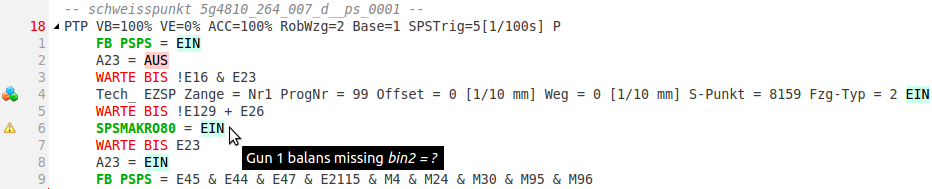Shown only if \$config.dat the file was loaded.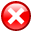The last syntax warnings' category
• Wrong VB value 0 <= VB <= 100
• Wrong VE value 0 <= VE <= 100
• Wrong Genau value 0 <= Genau <= 300
• Wrong ACC value 0 <= ACC <= 100

• Missing bracket
• Integer value exceed -2000000000 <= value <= 2000000000
• Comment text to long. Max 128 chars allowed
• Makro call not allowed for point with VE <> 0

• Wrong operand
• Too many operands
• Wrong operand number
• Wrong operand number 1 <= E <= 4096
• Wrong operand number 1 <= A <= 4096
• Wrong operand number 1 <= M <= 200
• Wrong operand number 1 <= F <= 999
• Wrong operand number 1 <= T <= 60
• Wrong operand number 1 <= S <= 32

• Wrong UP number! <= UP <= 999
• Wrong Folge number 1 <= Folge <= 999
• Wrong Makro number 0 <= Makro <= 999

• Wrong timer number 1 <= t <= 60
• Wrong SPSTrig value 0 <= SPSTrig <= 100
• Wrong label number 1 <= Label <= 32000
• Label not defined

• Single variable not defined
• Variable out of range 1 <= i <= 60
• Variable out of range 1 <= bin <= 64
• Variable out of range 1 <= t <= 60
• Variable out of range 1 <= ana <= 16
• Variable out of range 1 <= anain <= 16
• Variable out of range 1 <= binin <= 64
• Variable out of range 1 <= p <= 256

• Command not allowed in this type of point
• Command not allowed on this level
• Command not suported
• Command not suported in this file

• Command out of point fold
• Distance parameter for this point must be 0 [mm]
• Second FB ONL command not allowed
• Tech command not suported

• Undefined point motion type
• Wrong Base value 0 <= Base <= 32
• Wrong Tool value 0 <= Tool <= 32
• Inconsistent point data!
• Inconsistent base data in src and dat file. Base in dat file is...
• Inconsistent tool data in src and dat file. Tool in dat file is...
• Undefinied point motion type

• For suchlauf motion type must be LIN
• Wrong Fern value! Fern=[E|M]d+
• Wrong Nah value Nah=[E|M]d+
• Wrong Vred value Vred=[1-100]%

Others icons
•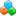informs the user that current command is VKRC technology command
•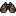informs the user that current point is SUCHLAUF motion